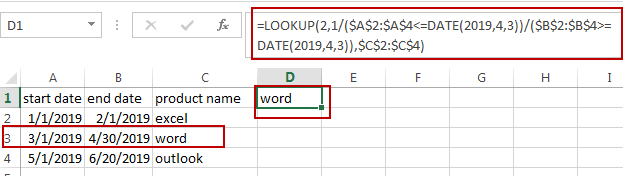# How to VLOOKUP to Return Value if Date Falls between Two dates in Excel

This post will guide you how to do a VLOOKUP between two dates and return corresponding value in Excel. How do I VLOOKUP between two dates and return corresponding value with lookup formula in Excel. How to lookup a value if the date fall between two dates in Excel.

## VLOOKUP Value If Date Falls between Two Dates

Assuming that you have a list of data in Range A1:C4, which contain start date, end date, and Sales product, and you want to lookup a given date (2019/4/3), if this date falls between the start dates and end dates, and then return its corresponding product name. How to achieve it. You can use a formula based on the LOOKUP function and the DATE function to achieve the result of doing vlookup operation to lookup a date and return value.

Like this formula:

`=LOOKUP(2,1/(\$A\$2:\$A\$4<=DATE(2019,4,3))/(\$B\$2:\$B\$4>=DATE(2019,4,3)),\$C\$2:\$C\$4)`

Type this formula into a blank cell, such as: D1, and then press Enter key in your keyboard. And the value will be returned in Cell D1.You just need to change the date number as you need in DATE function to do the VLOOKUP operation.

Video: VLOOKUP to Return Value if Date Falls Between Two Dates

### Related Functions

• Excel LOOKUP function
The Excel LOOKUP function will search a value in a vector or array.The syntax of the LOOKUP function is as below:= LOOKUP (lookup_value, lookup_vector, [result_vector])…
• Excel DATE function
The Excel DATE function returns the serial number for a date.The syntax of the DATE function is as below:= DATE (year, month, day)…

Related Posts

Calculate Compound Interest in Excel

This article will show you how to calculate compound interest in Excel. It will help you calculate the interest you will accrue on a given amount of money. There are several ways to calculate compound interest. One of the simplest ...

Count Attendance and Absence with COUNTIF function

In our campus life and work life, we usually record everyone's attendance. Today we will introduce you the application of Excel COUNTIF function to count the attendance. Using a week as an example, we use the following example to show ...

Find the Closest Data to the Data Provided in Excel

In our daily work, we may encounter such an issue that to find the closest value to a certain value. In fact, Excel internal functions can help us solve this problem. In today’s article, we will show you how to ...

Average Of Numbers With Multiple Criteria In Excel

Have you ever come across a task to calculate the average of the numbers with respect to multiple criteria? Are you tired of doing this cumbersome task manually? Are you willing to do this task smartly in just a matter ...

Calculate Average Of Last 5 Or N Values In Columns

Suppose you come across a task where you need to calculate the average of the last 2 or 3 numeric values, then what would you do? If you are new to Excel, then your first attempt might be doing this ...

Calculating Average Of The Numbers

Are you weary of investing a lot of time and effort in manually calculating the average of the numbers by including or excluding 0 and calculating the average of the top 3 scores? Then congratulations because you have just landed ...

Extract Unique Items From A List

This post will guide you how to extract unique itmes from a given list in Microsoft Excel. How to create a newly formula to get unique values from a range cells in Excel. The unique list of items is the ...

Average the Last N Numeric Values in Excel

AVERAGE function is one of the most popular functions in Excel. Apply AVERAGE together with some other functions, we can calculate average simply for some complex situations. In this article, we will introduce you to calculate average of the last ...

How to Find the First or Last Positive or Negative Number in a Column/List in Excel

Suppose we have a list of data entry, we want to look up the first positive number among them, is there any way to find it out? And if we want to look up the first or last negative number, ...

How to Find the Last or First Value in a Range Greater Than X in Excel

This post will guide you how to find the first value greater than a specified value in a range of cells in Excel. How do I find the last value greater that a given value in a row with a ...

Sidebar